# SAT Math Multiple Choice Question 160: Answer and Explanation

### Test Information

Question: 160

10. Ohm's Law, which can be written as IR = V, relates the current I in amperes that flows through a conductive material with resistance R ohms to the voltage V between the two ends. The power P in watts can be related to I and R by the equation I =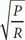. Which of the following gives P in terms of V and R ?

• A. p =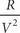• B. p =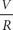• C. p =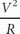• D. P = V2R3

C Whenever there are variables in the question and in the answer choices, think Plugging In, picking numbers that ensure that I is an integer. If P = 18 and R = 2, then I =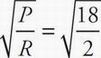=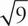= 3. Because V = IR, V = 3 × 2 = 6. Plug P = 18, R = 2, and V = 6 into the answers to see which answer works. Choice (A) becomes 18 =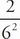. Solve the right side of the equation to get 18 =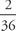. This statement is not true, so eliminate (A). Choice (B) becomes 18 =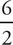. This statement is not true, so eliminate (B). Choice (C) becomes 18 =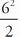. Solve the left side of the equation to get 18 =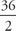. This statement is true, so keep (C), but check the remaining answer just in case. Choice (D) becomes 18 = (62)(23) or 18 = 36 × 8. This statement is not true, so eliminate (D). The correct answer is (C).